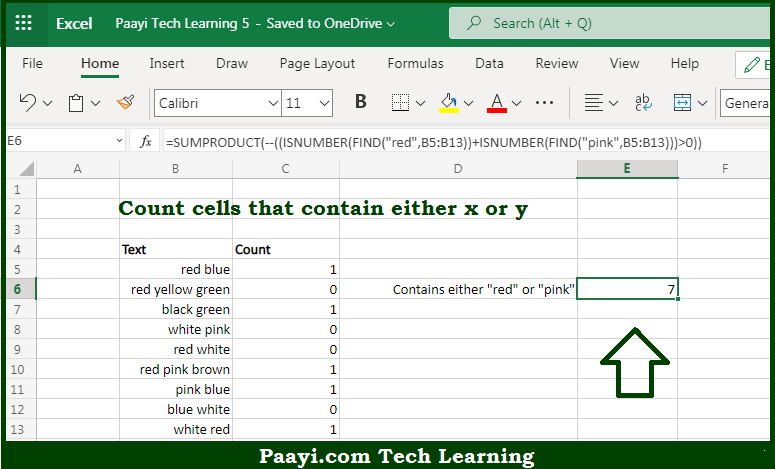# Learn How to Count Cells that Contain Either x or y in Microsoft Excel

Written by | 0 Comments | 607 Views

In this article, you will learn how to COUNT various things in Microsoft Excel using a single or combination of functions and its purpose in Microsoft Excel. You will also get to know how to count cells that contain either x or y & see the generic formula

Count Cells that Contain Either x or y in Microsoft Excel

The main purpose of this formula is to count cells that contain either x or y. Here we will learn how to count cells having either x or y in Microsoft Excel.  That implies, with the help of a formula based on the  SUMPRODUCT function you can able to count the number of cells that contain either x or y. So, with the help of this formula, you can able to count cells containing either x or y.

General Formula to COUNT Cells that Contain Either x or y

=SUMPRODUCT(--((ISNUMBER(FIND("abc",rng)) +  ISNUMBER(FIND("def",rng)))>0))

The Explanation for the COUNT Cells that Contain Either x or ySo we know that with the help of the given formula above you can able to count cells that contain either x or y. Here we will learn how to count cells having either x or y in Microsoft Excel. As we know that, when you count the cells using "OR logic", you need to be careful not to double count or recount. Let us understand this by the example, if you are counting cells containing "xyz" or "qwe", you don't need to just add together two COUNTIF functions, because you may double count cells that contain both "xyz" and "qwe". So, with the help of this formula, you can able to count cells that contain either x or y.